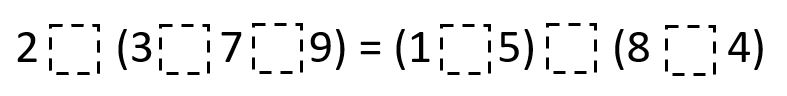Home > Grade 5 > Operation Symbols

# Operation Symbols

Directions: Use the operation symbols (+, -, x, and ÷) to make the equation true. Operation symbols may be used more than once.### Hint

What operations need to be done first?

There are multiple answers but one solution is 2 x (3 x 7 + 9) = (1 x 5) x (8 + 4) = 60

Source: Joshua Nelson

## Multiplying Fractions 4

Directions: Using the digits 1 to 9 at most once each time, fill the boxes …

1.This was very helpful and I would use this again

•it was helpful and help me very much

2.Good source!

3.I think it was a great way to get your mind started and it helps you get the answer and the work done positively and on paper.

4.I think its an amazing practice.

5.I came up with the same answer they did. It might also be helpful to try and make one side of the equation equal a nice round multiple of 10 first, then try to make the other side of the equation match.